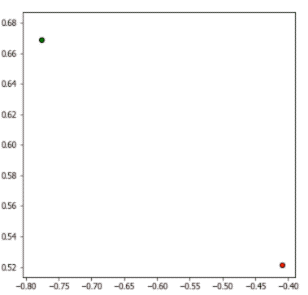# Anders Wang

### 用蒙特卡洛方法求圆周率π值

π值求解的推导公式如下：

$P(A)=\frac{四分之一圆面积}{正方形面积}=\frac{\frac{1}{4}πr^2}{r^2}=\frac{π}{4}$

$P(A) = \frac{π}{4}$

$π = P(A)\times4$import numpy as np
import pandas as pd
import math
import random
import matplotlib.pyplot as plt

random.seed(123)

# 总随机数量
total = 50000

# 区域内的数量
in_count = 0
x, y = [], []

# 红点x，y坐标
x_red, y_red = [], []
# 绿点x，y坐标
x_green, y_green = [], []

for i in range(total):
# random()方法默认为0，1边界。另外，由于演示的可视化为第二象限，所以x坐标取值为负数。
x.append(-random.random())
y.append(random.random())

# 也可以直接计算(x**2 + y**2)**0.5，因为开根号就等于0.5次方
distance = math.sqrt((x[i]**2 + y[i]**2))

if distance <= 1:
in_count += 1
x_red.append(x[i])
y_red.append(y[i])
else:
x_green.append(x[i])
y_green.append(y[i])

fig, ax = plt.subplots(figsize=(6, 6))
plt.scatter(x_red, y_red, c='red', edgecolor='black')
plt.scatter(x_green, y_green, c='green', edgecolor='black')

print(f'π: {4*in_count/total}')